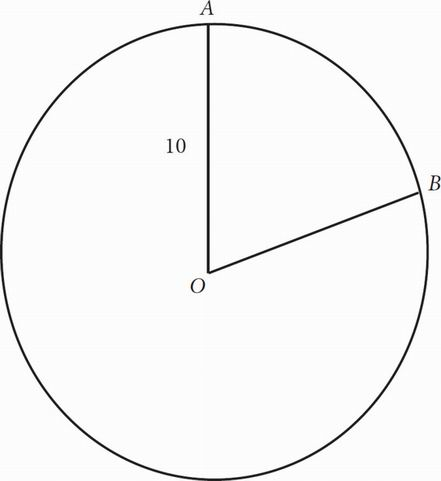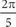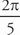# SAT Math Multiple Choice Question 158: Answer and Explanation

### Test Information

Question: 158

8.In the circle with center O and radius 10 shown above, ∠AOB =. What is the length of minor arc AB ?

• A. π
• B. 2π
• C. 4π
• D. 20π

C Use the formula arc = , where r is the radius and θ is the measure of the central angle in radians. Because the angle is already in radians, you just need to plug in 10 for the radius and the angleinto the formula. You then get s = (10)or 4π, which is (C).